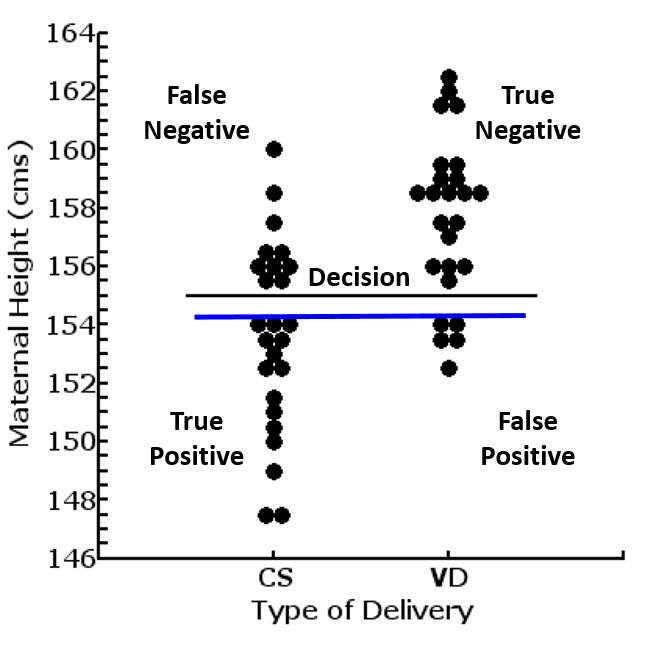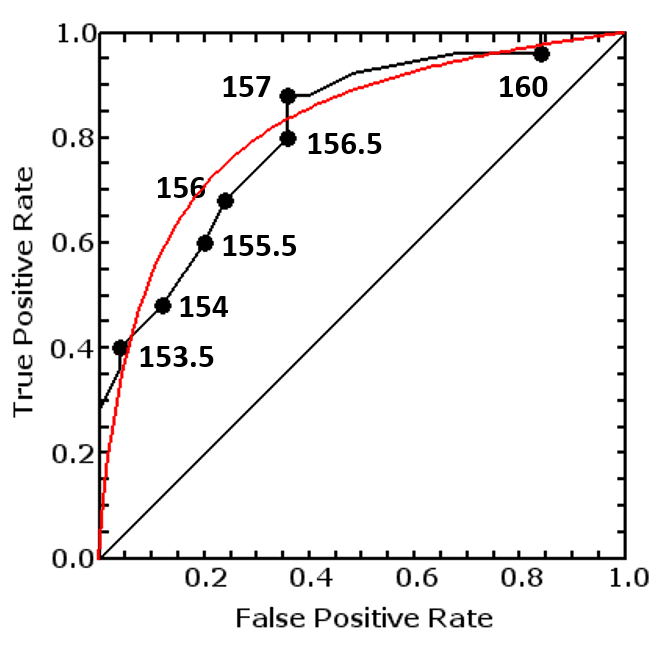Content DisclaimerCopyright @2020.All Rights Reserved.
StatsToDo : Curve Fitting for Receiver Operator Characteristics (ROCs) Explained
Introduction Example R Code
This page supports the program from the Curve Fitting for Receiver Operator Characteristics (ROCs) Program Page . The algorithm is adapted from that in a paper by Moses et.al. (see references)

The Need for Curve Fitting the Receiver Operator Characteristic (ROC) Curve

The underlying assumptions of the ROC curve is that the Outcome is binary, but the Test is a continuous measurement. In establishing the ROC from a set of reference data, it is assumed that the sample size provided is large enough, and the granularity of the values in the data set is fine enough, to produce a valid curve.

The modelling data set unfortunately often failed these assumptions. A major problem is that test measurements are often rounded to clinically meaningful intervals, so that the granularity of the data is coarse, and changes occurred in a series of steps rather than in a smooth curve.Furthermore, with rounding of values, often there are clusters with the same test value in one or both predictor groups, making interpretation difficult

• A large cluster will cause a large change in True and False Positive Rates, when the change in cut off value is small
• A cluster will result in an uneven relationship between false and true positive rates, as the values of one may change while the other remains the same.

An example of this is shown in the plot to the left. If the cut off for decision making is 155 (the black line), data points can be clearly separated into above or below that value. However, if the cut off value is set at 154, there are 3 data points in the Outcome Positive group and 2 in the Outcome Negative group that have this exact value. Whether these are considered above the cut off, below the cut off, or excluded from consideration, will have a large impact on subsequent results produced.The resulting ROC plot would be as the black line in the plot to the right.

Curve fitting would result in a ROC curve that has the closest fit with the data, yet allows a continuous interpretation along the whole range of the Test values, as shown in the red line in the plot to the right.

### References

Hanley JA, McNeil BJ (1982) The meaning and use of the Area Under a Receiver Operating Characteristic (ROC) curve. Radiology 143:29-36

Simel D.L., Samsa G.P., Matchar D.B. (1991) Likelihood ratios with confidence: sample size estimation for diagnostic test studies. J. Clin. Epidemiology vol 44 No. 8 pp 763-770

Moses LE, Shapiro D, and Littenberg B(1993) Combining independent studies of a diagnostic test into a Summary ROC Curve: Data analysis approaches and some additional considerations. Statistics in Medicine 12:p1293-1316.

Deeks J.J, and Morris J.M. (1996) Evaluating diagnostic tests. In Bailliere's Clinical Obstetrics and Gynaecology Vol.10 No. 4, December 1996 ISBN 0-7020-2260-8 p. 613-631.

Fagan T.J. (1975) Nomogram for Bayer's Theorem. New England J. Med. 293:257

Sackett D, Haynes R, Guyatt G, Tugwell P. (1991) Clinical Epidemiology: A Basic Science for Clinical Medicine. Second edition. ISBN 0-316-76599-6.